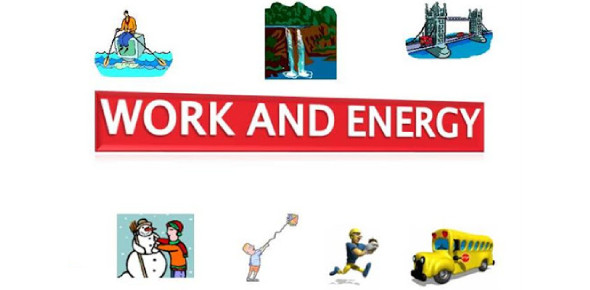# Work And Energy Quiz Questions And Answers

12 Questions | Attempts: 13447SettingsPlay this quiz, give answers to the questions and review your knowledge regarding work and energy. For work to take place, an amount of energy has to be used. Work can either change the kinetic energy or change the potential energy. Do you understand the connection between work and energy? Test how well you understood these two topics by taking the quiz below. All the best, and keep looking for more tests on the topic.

• 1.
What is the formula for work?
• A.

Force x Distance

• B.

Distance/Force

• C.

Force/Distance

• D.

None

• 2.
What is the relationship between Work, Force, and Distance?
• A.

Work is the what you get when a force acts over a certain distance. Therefore, without distance or force there would be no work.

• B.

Work and Distance are related because force is acted over a long distance.

• C.

Without work you do not have force.

• D.

All are true about work, force, and distance.

• 3.
What are the units for work?
• A.

Watts, and Joules

• B.

Dollars, and Euros

• C.

Newton Meter, and Joules

• D.

Watt and Joules

• 4.
If you exert a force of 800 newtons, and the object moves 10 meters how much work did you do?
• A.

8000 Joules

• B.

80 Joules

• C.

80,000 Joules

• D.

800 Joules

• 5.
What is energy, and how does it relate to work?
• A.

Energy is the ability to do work or make work, and without it work not is done.

• B.

Energy causes a change, and work is needed for energy to happen.

• C.

Energy is the ability to do work or cause a change, and work is the transfer of energy.

• D.

• 6.
What is the unit of measurement for energy?
• A.

Watts

• B.

Joules

• C.

Newtons

• D.

Killowatt

• 7.
What are the differences between Kinetic and Potential energy?
• A.

Kinetic energy is the energy of motion, and potential energy is stored energy

• B.

Potential energy is the energy of weight, and kinetic energy is the energy of nuclear atoms.

• C.

Potential energy is the energy of speed, and kinetic energy is the stored energy.

• D.

There is no difference between the two.

• 8.
Examples of Potential Energy are?
• A.

Gravielastic energy, and Joules

• B.

Gravitational potential energy which is dependent on the height of the object.

• C.

Nucleus potential energy, depending on the amount of nuclear power.

• D.

A person walking

• 9.
What is the formula for G.P.E, and do a calculation?
• A.

GPE= 1/2 Mass X Velocity 10kg x 54m/s = 540 540/2= 270

• B.

GPE= M x G x H 5kg x 10x 2m = 100 Joules

• C.

GPE= M x G x H 5kg x 10x 2m = 100 Watts

• D.

None

• 10.
What is the difference between Elastic Potential Energy and GPE?
• A.

GPE is potential energy that's dependent on height, EPE is energy associated with objects that can be stretched or compressed

• B.

GPE is potential energy dependent on weight only, and EPE is the potential energy only found in rubber bands.

• C.

GPE and EPE are the same, but are not written the same way.

• D.

Both are same as there is no difference between the two.

• 11.
What is the formula for Kinetic Energy?
• A.

1/2 Mass x Velocity squared

• B.

Mass x Velocity x 2

• C.

Velocity / Mass x 1/2

• D.

Mass x Velocity

• 12.
When is there more GPE in a swinging pendulum?
• A.

At the top

• B.

At its lowest point

• C.

In between the top, and the lowest point

• D.

At the bottom

## Related TopicsBack to top
×

Wait!
Here's an interesting quiz for you.